# Mathematical Introductions### An Introduction to Magic Squares

##### Age 7 to 16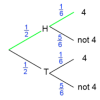### An Introduction to Tree Diagrams

##### Age 11 to 16

This article explains how tree diagrams are constructed and helps you to understand how they can be used to calculate probabilities.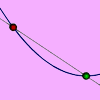### An Introduction to Differentiation

##### Age 14 to 18

An article introducing the ideas of differentiation.### An Introduction to Irrational Numbers

##### Age 14 to 18

Tim Rowland introduces irrational numbers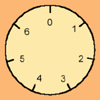### An Introduction to Modular Arithmetic

##### Age 14 to 18

An introduction to the notation and uses of modular arithmetic### An Introduction to Polar Coordinates

##### Age 14 to 18

This introduction to polar coordinates describes what is an effective way to specify position. This article explains how to convert between polar and cartesian coordinates and also encourages the creation of some attractive curves from some relatively easy equations.### An Introduction to Proof by Contradiction

##### Age 14 to 18

An introduction to proof by contradiction, a powerful method of mathematical proof.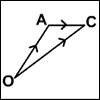### An Introduction to Vectors

##### Age 14 to 18

The article provides a summary of the elementary ideas about vectors usually met in school mathematics, describes what vectors are and how to add, subtract and multiply them by scalars and indicates why they are useful.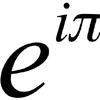### An Introduction to Complex Numbers

##### Age 16 to 18

A short introduction to complex numbers written primarily for students aged 14 to 19.### An Introduction to Galois Theory

##### Age 16 to 18

This article only skims the surface of Galois theory and should probably be accessible to a 17 or 18 year old school student with a strong interest in mathematics.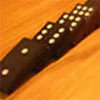### An Introduction to Mathematical Induction

##### Age 16 to 18

This article gives an introduction to mathematical induction, a powerful method of mathematical proof.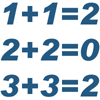### An Introduction to Mathematical Structure

##### Age 16 to 18

An introduction to the sort of algebra studied at university, focussing on groups.### An Introduction to Number Theory

##### Age 16 to 18

An introduction to some beautiful results in Number Theory.